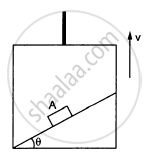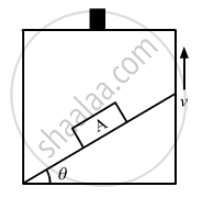# P a Block a Can Slide on a Frictionless Incline of Angle θ and Length L, Kept Inside an Elevator Going up with Uniform Velocity V in the Following Figure.. - Physics

Sum

A block A can slide on a frictionless incline of angle θ and length l, kept inside an elevator going up with uniform velocity v in the following figure. Find the time taken by the block to slide down the length of the incline if it is released from the top of the incline.#### SolutionThe force on the block which makes the body move down the plane is the component of its weight parallel to the inclined surface.
F = mg sinθ
Acceleration, g = sin θ
Initial velocity of block, u = 0
Distance to be covered
s = l
a = g sin θ

Using, $s = ut + \frac{1}{2}a t^2$

$l = 0 + \frac{1}{2}\left( g\sin\theta \right) t^2$

$\Rightarrow t^2 = \frac{2l}{g\sin\theta}$

$\Rightarrow \text{ Time taken }, t ={\sqrt{\frac{2l}{gsin\theta}}}$

Concept: Newton’s Second Law of Motion
Is there an error in this question or solution?

#### APPEARS IN

HC Verma Class 11, Class 12 Concepts of Physics Vol. 1
Chapter 5 Newton's Laws of Motion
Q 40 | Page 83